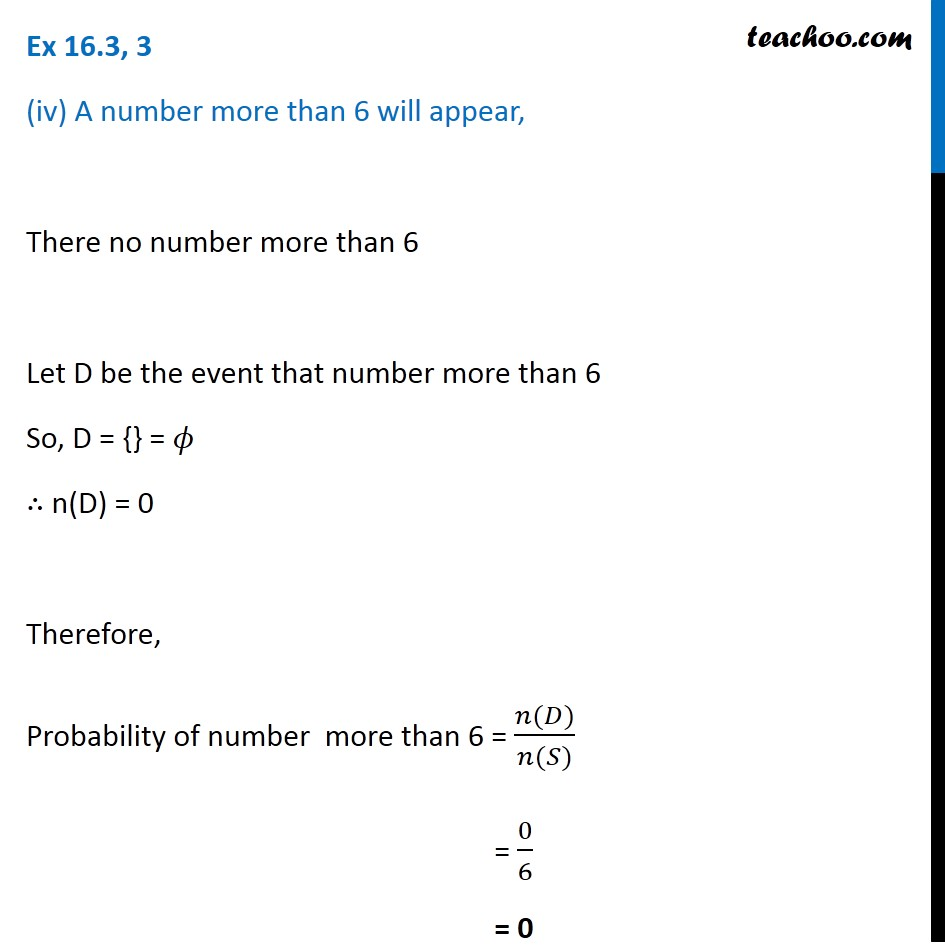Ex 14.2

Chapter 14 Class 11 Probability
Serial order wiseLearn in your speed, with individual attention - Teachoo Maths 1-on-1 Class

### Transcript

Ex 14.2, 3 (iv) A number more than 6 will appear, There no number more than 6 Let D be the event that number more than 6 D = {} = 𝜙 n(D) = 0 Probability of number more than 6 = (𝑛(𝐷))/(𝑛(𝑆)) = 0/6 = 0